# Kerala Syllabus 10th Standard Physics Solutions Chapter 5 Refraction of Light

## Kerala State Syllabus 10th Standard Physics Solutions Chapter 5 Refraction of Light

### Refraction of Light Textual Questions And Answers

Textbook Page No. 103

Sslc Physics Chapter 5 Kerala Syllabus Question 1.
Do we observe the objects underwater at their original position?
No.

Sslc Physics Chapter 5 Notes Kerala Syllabus Question 2.
Observe the path of light. Do you observe anything out of the ordinary? Can you de¬pict it in the science diary?
The light rays below the water undergo deviation at the surface of water.

Textbook Page No. 104

Refraction Of Light Class 10 Kerala Syllabus Question 3.
Which are the media involved here?
Air & Water.

Sslc Physics Refraction Of Light Kerala Syllabus Question 4.
What happens to the path of the light?
Path of light undergoes deviation.

Sslc Physics Chapter 5 Refraction Of Light  Question 5.
Where does the deviation of the ray take place?
At surface of water.

Physics Class 10 Chapter 5 Kerala Syllabus Question 6.
Why does the ray of light undergo a deviation here?
The difference of speed of light rays in different media.

Physics Chapter 5 Class 10 Kerala Syllabus Question 7.
Does light pass through all the media at the same speed?
No

Class 10 Physics Chapter 5 Kerala Syllabus Question 8.
As the optical density of a medium increases, the speed of light through it decreases.
What if the optical density decreases?
Speed of light increases.

Class 10 Physics Chapter 5 Notes Kerala Syllabus Question 9.
Can the media given in the table be arranged in the increasing order of their optical densities?
Air<……….. <……… <……….
Air < Water < Glass < Diamond

Textbook Page No. 105

10th Class Physics Chapter 5 Notes Kerala Syllabus Question 10.
Which is the incident ray on the surface of separation CD?
QR

Refraction Of Light Class 10 State Board Kerala Syllabus Question 11.
The angle between the incident ray and the normal is called the angle of incidence. If so, can you explain what is angle of refraction??
∠r Between the refracted ray and the normal

Class 10 Physics Chapter 5 Kerala Syllabus Question 12.
Is the angle of refraction greater or lower than the angle of incidence when it goes from air to glass?
Lower

Sslc Physics Chapter 5 Questions And Answers Kerala Syllabus Question 13.
What about from glass to air?
Greater

Chapter 5 Physics Class 10 Kerala Syllabus Question 14.
Which is of greater optical density – air or glass?
Glass

Physics Chapter 5 Class 10 Notes Kerala Syllabus Question 15.
While going from air to glass, the refracted ray deviates towards the normal/deviates away from the normal.
Deviates towards the normal. While entering from air to glass (from a medium of lower optical density to that of a greater one) the refracted ray deviates away from the normal.

Textbook Page No. 106

Chapter 5 Physics Class 10 Notes Kerala Syllabus Question 16.
What happens while it goes from glass to air?
Deviates away from the normal. Normal. While entering from glass to air (from a medium of greater optical density to that of a lower one) the refracted ray deviates away from the normal.

Physics Class 10 Refraction Of Light Solution Kerala Syllabus Question 17.
Are the angle of incidence, angle of refraction and the normal at the point of incidence on the same plane?
Yes. The angle of incidence, angle of refraction and the normal at the point of incidence are in the same plane.

10th Class Physics Chapter 5 Kerala Syllabus Question 18.
Does refraction take place for a ray while entering a glass slab normal to it?
No

Sslc Physics Chapter 5 Solutions Kerala Syllabus Question 19.
Examine using a ray of light from a laser torch. Ray diagrams of a light ray passing through different media are depicted. Find out the appropriate figures by observing these figures and also based on the concepts you have developed from the textbook.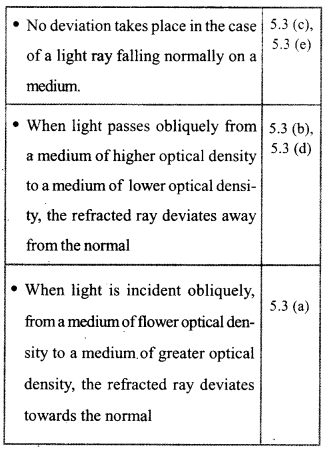Textbook Page No. 107

Sslc Physics Chapter 5 Notes Pdf Kerala Syllabus Question 20.
Its an experiment, can you find out the path of light through a triangular prism using a laser torch? Record it in the science diary.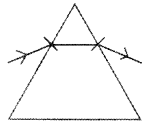10th Class Physics Refraction Of Light Kerala Syllabus Question 21.
What change occurs in the angle of refrac¬tion with the increase in the angle of inci¬dence when a ray enters a medium?
Increases

Physics 10th Class Chapter 5 Kerala Syllabus Question 22.
Can you analyze the table and find out the change? What other conclusions can you arrive at from the table?
When light passes through different pairs of media, the angle of refraction increases with the angle of incidence. The ratio of the sine of the angle of incidence to the sine of the angle of refraction $$\left(\frac{\sin i}{\sin r}\right)$$ will be a constant. This constant is known as refractive index.

Textbook Page No. 108

Reflection Of Light Class 10 Kerala Syllabus  Question 23.
What specialty is observed in the value of ratio of sine of the angle of incidence to the sine of the angle of refraction, $$\left(\frac{\sin i}{\sin r}\right)$$?
Will be a constant.

Textbook Page No. 109

Physics Class 10 Chapter 5 Notes Kerala Syllabus Question 24.
What will be refractive index n12? Refractive index, n12 =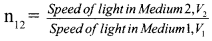Textbook Page No. 110

Question 25.
Complete the table 5.6 (a)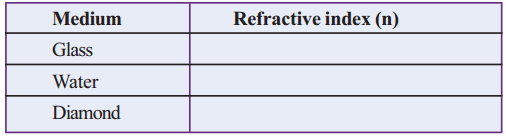Question 26.
If the speed of light in water is 2.25 x 108 m/s
a. Calculate the speed of light in vacuum
b. Calculate the speed of light in glass.
a. Speed of light in vacuum = Speed of light in water × Refractive index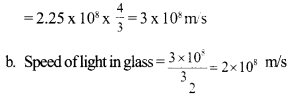Question 27.
Place a pencil in an inclined position in a glass trough and fill three fourth of the trough with water. Depict in your science diary, the changes after adding water. What shall be the reason for the change?
Don’t you see that the position of the portion of the pencil underwater has changed? What may be the reason? Discuss.The light rays from pencil undergo deviation at the surface of water.

Textbook Page No. 111

Question 28.
Does the ray of light coming after reflection from the pencil undergo a deviation? What is the reason?
Due to refraction

Question 29.
Now, will you be able to explain why a frog was obtained through the arrow was aimed at the fish?
Light rays from fish and frog undergo deviation at surface of water. So they don’t see on exact place.

Question 30.
What is observed here?
The coin seems to be lifted up.

Question 31.
What is the reason for this observation?
As light from the coin comes from denser to rarer medium if bends away from normal so the position of the coin seems to be lifted up due to refraction.

Question 32.
Draw a thick line on a paper using a pen. Place a glass over it and observe as suggested below.
a Look from one side as shown in Fig. 5.8 (a)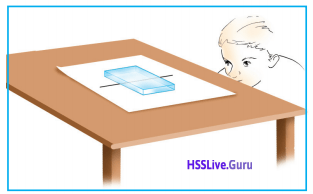b. Look from one side as shown in Fig. 5.8(b)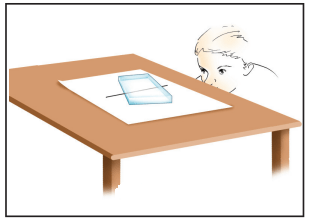c. Look from one side as shown in Fig. 5.8 (c)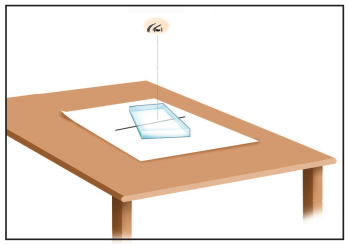a. The line seems to be rising on glass slab.
b. The line seems to be the surface of glass slab.
c. The line seems to be below the glass slab.

Textbook Page No. 112

Question 33.
Can you easily pick up the coin?
We can’t easily pick up the coin.

Question 34.
What is the reason for the failure?
Because coin’s actual place is not there. The light rays from the coin comes from denser to rarer medium it bends away from the normal, So the position of coin seems to be lifted up due to refraction.

Question 35.
Find out more examples of refraction from daily life.
The stars in the sky appear glittering

• We can see the sun when it falls below the horizon.
• The bottom of water appears raised.
• We cannot locate the position of fish in the water

Question 36.
A person who looks at an aquarium as shown in Fig. 5.10 can see the base on the surface of water. What is the reason?
The light rays from the bottom of the aquar¬ium reflect without undergoing refraction (Due to total internal reflection)

Textbook Page No. 113

Question 37.
What will be the angle of refraction when the refracted ray passes along the surface of water?
Angle of refraction to becomes 90°.

Question 38.
Allow light to fall at an angle of incidence greater than the critical angle. What do you observe?
The ray is reflected back to the same medium without undergoing refraction.

Textbook Page No. 114

Question 39.
Which are the figures that show total internal reflection?Question 40.
What is the critical angle of glass?
42°

Question 41.
Will total internal reflection take place when light passing through water is inci¬dent on the surface of separation with air at an angle of incidence of 45°? Why?
No. The critical angle of water 48.6°. You have already realized that total internal re-flection takes place only if the angle of incidence is greater than the critical angle. The total internal reflection takes place when in the incident ray is more than 48.6°

Question 42.
Find out the practical applications of total internal reflection in our day to day life.
1. Medical field – Endoscope
2. In the field of communication – Optical fiber cables.
1. In the field of treatment- In the endoscope
2. In the field of telecommunication, optical fiber cables are used here.
3. Prism is used in the binoculars
4. Prism is used in the periscopes instead of mirrors.

Textbook Page 117

Question 43.
Complete the table 5.7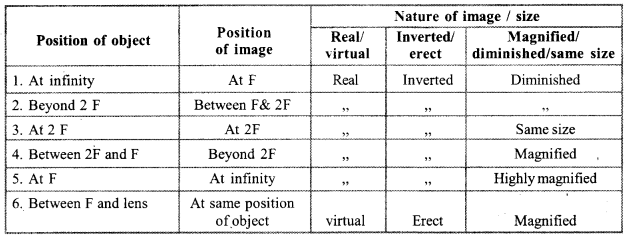Textbook Page 119

Question 44.
Where will the rays coining parallel to the principal axis converge?
At principal focus

Question 45.
Where is the image formed?
At principal focus

Question 46.
Write down the characteristics of the image.
Position of the image: Between F and 2F
Nature of the image: Real, Inverted
Size of the image: Diminished

Textbook Page 120

Question 47.
Object at 2F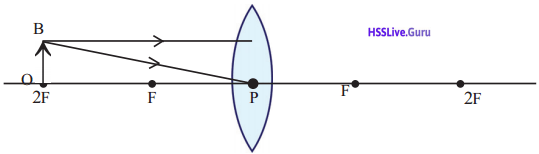Position of the image: At 2F on the other side Characteristic of the image: Inverted Size of the image: Same size of the object.

Question 48.
Object between F and 2F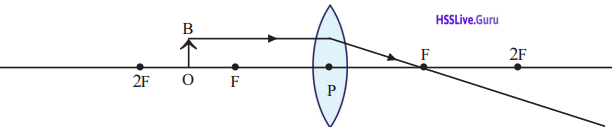Position of the image: Beyond 2F
Characteristic of the image: Inverted
Size of the image: Bigger than the object

Question 49.
Object at F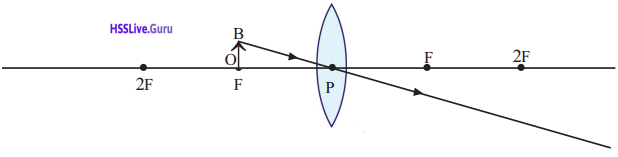Position of the image: At the infinity
Characteristic of the image: Inverted
Size of the image: Big

Textbook Page 121

Question 50.
Object between F and LensPosition of the image: On the same side of the object
Characteristic of the image: direct image
Size of the image: Big in size

Question 51.
Have you observed images formed by a concave lens? What is the nature of the image?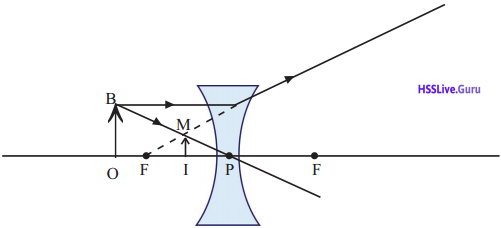Images is smaller, virtual and direct

Textbook Page 122

Question 52.
Record the measurement shown in the figure as per the Cartesian System.a. Distance of the object from the lens (u) = ………….
b. Distance of the image from the lens (v) = ………….
c. Height of object (OB) = …………..
d. Height of image (IM) = …………..
a. u = -25cm
b. v = + 100cm
c. OB = +1cm
d. IM = -4 cm

Question 53.
Complete the Table 5.8Average f = 19.99 cm

Textbook Page 123

Question 54.
Is there any relation between the height of an object and the height of its image?
Can it be related to the ratio between the distance to the object and that to the image?
The height of the object height of the image depends on the ratio of the distance to the object and distance to the image. When the object is kept at different positions, the height of the image can be varied.

Question 55.
When an object is placed at different positions in front of a lens, isn’t there a change in the height of the image?
Yes

Question 56.
Calculate the magnification of the image formed by convex lens in Fig. 5.33
Height of the image = (hi) = -4 cm
Height of the object = (h0) = +1 cm
Magnification = $$\frac{h_{1}}{h_{0}}=\frac{-4}{+1}=-4$$
or in another way
m = $$\frac { v }{ 4 }$$
v = +100, u = -25
m = $$\frac { 100 }{ -25 }$$ = -4

Textbook Page 125

Question 57.
Find out the uses of lenses in our day to day life and record them in the science dairy.

• In telescope
• In eye lenses
• In-camera
• In the microscope
• In the magnifying a lenses

Question 58.
What has the doctor indicated in the prescription?
The doctor indicated about the type and power of lens.

Question 59.
Calculate the power of a lens of focal length + 25 cm.Question 60.
You can guess what the +2D in the prescription stands for. What is its focal length? What kind of lens is it?
p = + 2D
It is a convex lense
f = $$\frac { 1 }{ p }$$ $$\frac { 1 }{ 2 }$$ = 50cm

Question 61.
You might have seen the twinkling of stars at night. But planets do not twinkle. Why?
Light coming from distant stars passes through different layers of air. Each layer differs from the other in their optical densities. Hence light undergoes successive refraction. Since stars, at a greater distance, they appear like a point source. The rays of light appear to come from different points on reaching the eye after refraction. This is the reason for the twinkling of stars.

Let Us Assess

Question 1.
Refractive indices of different materials are given. Find out the medium through which light passes with maximum speed.
Water

Question 2.
The nature of images formed by two lenses are given.
i. An erect and magnified virtual image.
ii. An erect and diminished virtual image
a. What type of lens is used in each case?
b. By using which type of lens will we get an image having the same size as the object? What is the position of the object?
a. i. Convex lense
ii. Concave lense
b. Convex lense. Object will be at 2F.

Question 3.a. MN represents a lens. What type of lens is this?
b. What are the characteristics of the image?
c. Copy the ray diagrams in the science diary and complete it
a. Convex
b. Magnified, real, invertedQuestion 4.
What do you mean by power of a lens? What is the SI unit of the power of a lens? Calculate the power of a concave lens of focal length 25 cm.
Power is a term related to the focal length of a lens. Power of a lens is the reciprocal of focal length expressed in meters. Power
p = 1/f
Unit of power is dioptre. It is represented byQuestion 5.
Observe the figure. Light falling on two different media are shown.a. Which medium has greater optical density? Why?
b. Which medium has greater refractive index?
a. Medium 1. Angle of incidence is same, but angle of refraction is different. When a ray of light passes from a medium of lower density to greater density the refracted ray deviates towards the normal. Medium 1 has lowest angle of refraction compared to medium 2.
b. Medium 1

Question 6.
An object of height 3 cm is placed in front of a convex lens of focal length 20 cm at a distance of 30 cm.
a. What is the distance to the image formed?
b. What is the nature of the image?
c. What is the height of the image?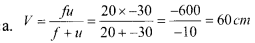b. Magnified, inverted, realQuestion 7.
In the table, the absolute refractive indices of certain transparent media are given.

 Medium Refractive index Air 1.0003 Water 1.33 Kerosene 1.44 Turpentine oil 1.47 Crown glass 1.52 Diamond 2.42

a. Find out from the table the medium of highest and lowest optical densities,
b. If the speed of light in air is 3 x 108 m/s, what will be the speed of light through kerosene?
c. W ill a ray of light deviates towards the normal or away from the normal when it enters from air to diamond obliquely?
d. The refractive index of diamond is 2.42. What do you mean by this? Calculate the speed of light through diamond.
a. Highest – Diamond
Lowest – air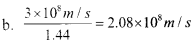c. Towards the normal
d. The speed of light in air (Vaccum) is 2.42 times greater than the speed of light in diamond or other hands. Speed of light in diamond is 2.42 times lesser than speed of light in air or vacuum.
Speed of light through diamond
$$=\frac{3 \times 10^{8}}{2.42}=1.25 \times 10^{8} \mathrm{m} / \mathrm{s}$$

Extended Activities

Question 1.
Half portion of a convex lens is wrapped with a black paper. Can this lens give a complete real image of an object? Explain.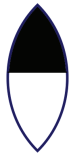Yes. This lens gives a complete real image of an object. However, the intensity of image may be less.

Question 2.
The refractive indices of different media are given. Analyze the table and answer the following.
In which medium will light have the highest speed?
Water

Question 3.
Glycerine, water and sunflower oil are taken in two beakers. A glass rod is dipped in one and a pyrex glass rod is dipped in the other. Do they appear in the same way? In which media are they visible? Justify.Objects are visible because they reflect some of light that shines on them. Refractive index of glycerine, sunflower oil, and pyrex glass are same. Glass rod clearly visible in them. But pyrex glass is not clearly visible because light ray are passed without deviation through medium having same refractive index.

Question 4.Take a clean mineral water bottle and fill it with water. Put a hole on one side of the bottle. Allow water to flow out while passing a laser ray through it What do you observe? Why?
As water throws out of the opening observe the case beam remains trapped in the water stream because of total inemal reflection. As light tries to pass from the more dense water to the less dense air. it bends. In narrow column of water, the light wave continues bouncing off the boundaries of the stream of water but cannot pass through into the air.

### Refraction of Light Exam Oriented Questions & Answers

Very Short Answer Type Questions (Score 1)

Question 1.
The power of a convex lens of focal length 2 m is …………..
$$P=\frac{1}{f}=\frac{1}{2}=0.5 \text { dioptre }$$

Question 2.
What change occurs for power of a lens when it’s focal length increases?
Power decreases

Question 3.
An object is placed at 2F in the case of a convex lens what is its magnification? (greater than 1, less than 1, zero, one)
one

Question 4.
Figure shows a ray of light obliquely travels from air to glass. Find out the correct one.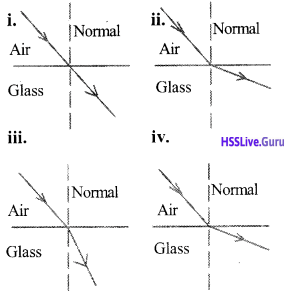Figure iii
It bent towards the normal

Question 5.
Find out the relationship in the first pair and fill in the second pair appropriately.
Focal length – meter
Power of the lens – …………..
Dioptre

Very Short Answer Type Questions (Score 2)

Question 6.
The image is direct and is above principal axis an object is kept at a distance of 30 cm away from a lens. An image of same size is formed. What is the focal length of the lens?
Image of size is formed when the object is kept at 2F
∴ 2f = 30 cm
∴ f = 15 cm
∴ $$\frac { 30 }{ 2 }$$ = 15cm

Question 7.
Write two situations when angle of refraction is 90°
i. The angle of incidence should be equal to critical angle.
ii. Rays of light should travel from the medium of greater optical density to lesser optical density

Question 8.
Represent the image formation by a concave lens. Why the focus of this lens is called virtual?The rays of light pass through a concave lens do not really meet. They appear to meet. So the focus is called virtual.

Question 9.
What are the situation where total internal reflection take place?
i. Rays of light travel from medium of greater optical density to that of lesser one.
ii. Angle of incidence should be greater than critical angle.

Question 10.
An object ‘OB’ is placed in front of lens is given below.a. Name the lens?
b. Complete the figure, then find out the position of the image.
c. Identify the two nature of the image.
a. Convex lensImages formed in between F and 2F.
b. Real and inverted images formed.

Question 11.
Complete the figure then find out the principal focus of the concave lens.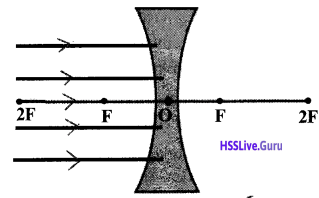Question 12.
Terms related to lens are given below? Complete the questions using these terms?
(Pole, Focal length, Centre of curvature, Principal axis)
a. The midpoint of a lens is called …………..
b. The distance between pole to focus is called ……………
c. The center of imaginary spear of two sides of the lens is called ……………
d. Imaginary line passing through the center of curvature of the lens is called …………..
a. Pole
b. Focal length
c. Centre of curvature
d. Principal axis

Question 13.
Corrected the wrong statement from the following.
a. Optical density of two mediums are different, it is reason for refraction.
b. Velocity of light is very high in optically denser medium.
c. Optical density of glasses lower than that of water
d. Velocity of light in vacuum is 3 × 108 m/s
Wrong statements – b, c
b. Velocity of light is comparatively low in optically denser medium.
c. Optical density of glasses higher than that of Water.

Question 14.
Calculate the power of the lens of focal length 10 cm?
f = 10 cm = 0.1 m
$$P=\frac{1}{f}=\frac{1}{0.1}=+10 \text { dioptre }$$

Question 15.
‘IM’ is an image of the object ‘OB’. Find out the given values related to new cartesian sign conventions.a. Distance between lens to object (u) ……………..
b. The distance between lens to image (v) …………
c. Height of the object (OB) ………….
d. Height of the image (IM) …………
a. u = -40 cm
b. v = +24 cm
c. OB = +2 cm
d. IM = -1cm

Question 16.
The statements associated with a lens are given by the new cartesian symbol. Write the correct one?
a. All distance are measured from the focus.
b. All distances measured along the direction of incident light is positive.
c. Lightray is assumed to travel from right to left.
d. X-axis is taken as the origin.
b. All distances measured along the direction of incident light is positive
d. X-axis is taken as the origin

Short Answer Type Questions (Score 3)

Question 17.
Observe the figure. Light rays enter from medium 1 to medium 2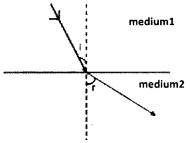a. Which medium has greater optical density?. How do you reach in such a conclusion?
b. How do optical density and velocity of light related?
c. What is the speed of light in vacuum?
a. medium 1
When the light rays enter from medium 1 to 2, they more away from the normal. The speed of light is also greater in medium 2 then medium.
b. When optical density increases the speed of light decreases.
c. 3 × 108 m/s

Question 18.
A virtual image of an object is formed at 30 cm away from a lens when an object is kept at a distance 20 cm away from it. What is the focal length of the lens? Find the magnification of the image?
u = -20 cm, v = -30 cm
(as the image is virtual, it is formed at the same side)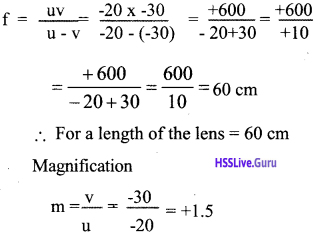Question 19.
It is written by the doctor for the eye lens as -2.50.
a. What the defect of eye of the patient?
b. What is the focal length of the lens?
c. What type of lens is it?
a. near sightedness
b. p = -2.5 D
$$\mathrm{f}=\frac{1}{\mathrm{p}}=\frac{1}{2.5}=\frac{-10}{25}$$
= -0.4 m = -40 cm
The focal length of the lens = – 40 cm
c. Concave lens

20. Observe the figure given below.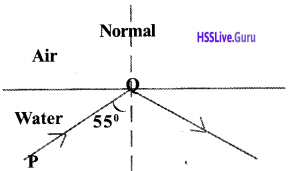a. Why the ray PQ reflected back as in the above figure?
b. Name this phenomenon?
c. What happens to the ray of light when angle of incident is 30°.
a. Angle of incidence is greater than that of critical angle.
b. Total internal reflection
c. Refraction takes place when it travels from water to air.

Question 21.
Nature of some images are given below. Find out the real and virtual images and then write it in separate column.
a. Inverted image
b. Do not obtained on a screen
c. Obtained on a screen
d. If the light rays really intersect to each other.
e. Erect image
f. The image distance cannot directly
Real images: a, c, d
Virtual images: b, e, f

Question 22.
A burning candle is placed in front of a convex lens to obtain an image on the screen.
Find out the positions of the object in each of the conditions given below?
a. We get the size of the image is equal to the size of the object.
b. The size of the image is smaller than that of the object.
c. We get large real image than the object.
a. 2F or C
b. Beyond 2F
c. In between 2F and F.

Question 23.
A person uses a spectacle of power of lens -1.25D.
a. What type of lens is this?
b. What is meant by power of a lens?
c. Find the focal length of the lens?
a. Power of the lens is negative. So it is a concave lens.
b. Power of a lens is the reciprocal of its focal length.Question 24.
Observe the figure carefully OB is an object placed in front of the convex lens.a. Complete the ray diagram then find out the position of the image.
b. An object is placed at 2F, in which position image is formed?
c. Where we have to place an object to get a virtual image from a convex lens?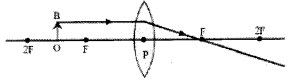a. Beyond 2F
b. Image is formed at 2F
c. An object is placed in between optic center and focus

Short Answer Type Questions (Score 4)

Question 25.
Combine suitable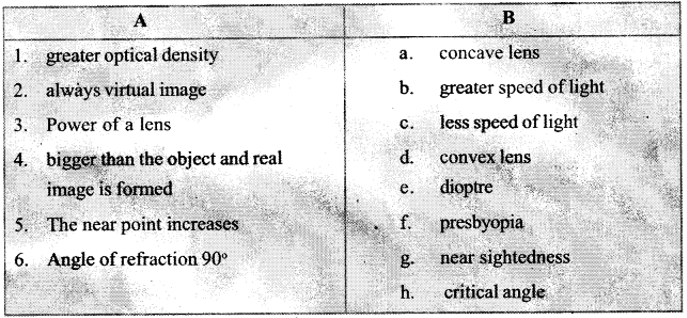1 – (c)
2- (a)
3 – (e)
4 – (d)

Question 26.
Light obliquely travels from glass to air, critical angle of Glass is 42°.
a. What is mean by critical Angle?
b. Angle of incidence is 42° find its refracted angle?
c. In which phenomenon we observe the incident angle is 40°? Explain the phenomenon?
d. What happens to the ray of light when the angle of incidence is 45°? Explain the phenomenon?